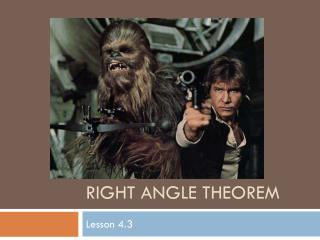DownloadDownload PresentationRight Angle Theorem

# Right Angle Theorem

Download Presentation## Right Angle Theorem

- - - - - - - - - - - - - - - - - - - - - - - - - - - E N D - - - - - - - - - - - - - - - - - - - - - - - - - - -
##### Presentation Transcript

1. Right Angle Theorem Lesson 4.3

2. Theorem 23:If two angles are both supplementary and congruent, then they are right angles. 2 1 Given: 1 2 Prove: 1 and 2 are right angles.

3. Paragraph Proof: Since 1 and 2 form a straight angle, they are supplementary.Therefore, m1 + m2 = 180°. Since 1 and 2 are congruent, we can use substitution to get the equation: m1 + m2 = 180° or m1 = 90°. Thus, 1 is a right angle and so is 2.

4. Given: Circle P S is the midpoint of QR P Prove: PS QR Τ S Q R • Circle P • Draw PQ and PR • PQ  PR • S mdpt QR • QS  RS • PS  PS • PSQ  PSR • PSQ  PSR • PSQ & PSR are supp. • PSQ and PSR are rt s • PS QR • Given • Two points determine a seg. • Radii of a circle are  . • Given • A mdpt divides a segment into 2  segs. • Reflexive property. • SSS • CPCTC • 2 s that make a straight  are supp. • If 2 s are both supp and , they are rt s. • If 2 lines intersect to form rt s, they are . Τ Τ

5. Given: ABCD is a rhombus AB  BC  CD  AD Prove: AC BD A D 5 4 7 2 E 1 Τ 3 6 8 B C Hint: Draw and label shape! • Given • Reflexive Property • SSS • CPCTC • If then • ASA • CPCTC • 2 s that make a straight  are supp. • If 2 s are both supp and  they are rt s. • If 2 lines intersect and form rt s, they are . • AB  BC  CD  AD • AC  AC • BAC  DAC • 7  5 • 3  4 • ABE  ADE • 1  2 • 1 & 2 are supp. • 1 and 2 are rt s • AC BD Τ Τ